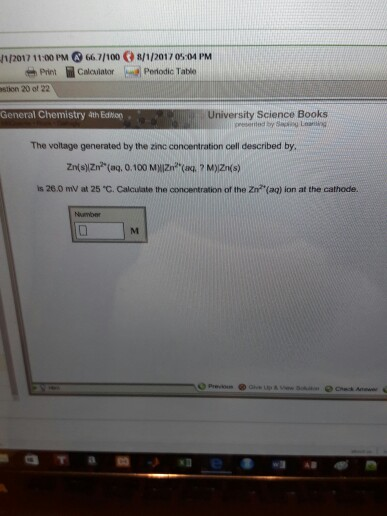# Question & Answer: The voltage generated by the zinc concentration cell described by, Zn(s)Zn^2+ (aq,…..The voltage generated by the zinc concentration cell described by, Zn(s)Zn^2+ (aq, 0.100)||Zn^2+ (aq M) Zn(s) is 26.0 mV at 25 degree C. Calculate the concentration of the Zn^2+(aq) ion at the cathode. Number M

Number of electron being transferred in balanced reaction is 2

Don't use plagiarized sources. Get Your Custom Essay on
Question & Answer: The voltage generated by the zinc concentration cell described by, Zn(s)Zn^2+ (aq,…..
GET AN ESSAY WRITTEN FOR YOU FROM AS LOW AS \$13/PAGE

So, n = 2

Since this is concentration cell, Eo = 0.0. V

Use:

E = Eo – (0.0592/n) log {[Zn2+]^1/[Zn2+]^1}

0.026 = 0.0 – (0.0592/2) log (0.100/[Zn2+])

log (0.1/[Zn2+]) = -0.8784

(0.1/[Zn2+]) = 0.1323

[Zn2+] = 0.756 M